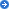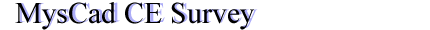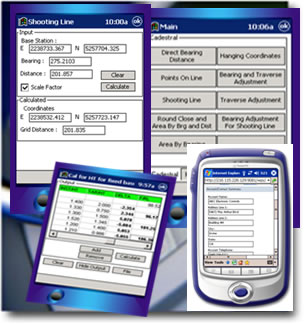NewsNew HYDRO MAPPER released!

Reseller Wanted
Phone: (60)-082-414751
Fax: (60)-082-416751

Events2014 : XXV International Federation Of Surveyors Congress (FIG 2014)Philippines NLAA Event 2011Philippines MAA Event 2011The 11th South East Asian Survey CongressMap Asia 2010 - KLCCMap Asia 2009 - SingaporeMap Asia 2008 - KLCCCollege Sarawak Career Fair 2008 (July)College Sarawak Career Fair 2008 (March)International Symposium & Exhibition on Geoinformation 2007Map Asia 2007The 1st Malaysian Software Engineering ConferenceKOJUTA 5th Annual MeetingInternational Symposium & Exhibition on Geoinformation(ISG) 2005

CounterHome > Products > MysCad CE SurveyMore Sample

MysCad CE Survey (SurcompCE) encompass all the cogo functions that required for the survey computation. It render conveniency as it is designed for the palm top which is portable to wherever the operators go.

## Features:

• Calculation of RSO meters coordinates from given latitude and longitude.
• Calculation of latitude and longitude from given RSO meters coordinates.
• Calculation of zero constant for short range edit using 21 measurements test rays.
• Calculation of bearing from a station within Sarawak to Mecca.
• Calculation for combined bearing and coordinate adjustment by "Weighted Means" for divisional control traverse.
• Calculation for combined bearing and coordinates adjustment for STG and above, where new starting and closing traverse leg bearing is held fixed.
• Calculation for combined bearing and coordinates adjustment by "Weighted Means" for STG and above.
• Calculation of identification of star for calculation of azimuth by star.
• Calculation of zenith angle and azimuth of reference object station (RO) for azimuth by star.
• Calculation of reduced level for nodal station by "weighted Means“.
• Calculation for adjustment for traverse, using prismatic compass bearing.
• Calculation of RSO coordinates for traverse by bearing swing method, where starting and ending stations coordinates in RSO and Cassini known.
• Calculation of station coordinates on circular curve, where spacing between station is equal that is equal cord.
• Calculation of station coordinates on circular curve with running chainage.
• Calculation of all stations coordinates, bearing and distance with running chainage for circular curves, spiral curves or combined circular and spiral curve.
• Calculation of stations coordinates, bearing and distance with running chainage form starting station, for curve with transition spiral curve.
• Calculation of direct bearing and distance between two stations, from fixed base to radiant rays and for traverse for Cassini project.
• Calculation of intersection station coordinates form four given known stations.
• Calculation of reduced level of an inaccessible station from two known base.
• Calculation of direction and distance or bearing, distance and coordinates to inaccessible centre of circular object by measurement of circumference and two rays observations.
• Calculation of station coordinates on semi-circle on line joining two given base.
• Calculation of arc-chord from fixed base to radiant rays to bring observation to plane.
• Calculation of orientation bearing based on one line given bearing.
• Calculation for location of distant stations from a given base station.
• Calculation of convergence from given RSO meters coordinates.
• Calculation of arc-chord for traverse to bring observation to plane.
• Calculation for reduction of Telcurometer Measurements.
• Calculation for eccentric reduction to centre for observation.
• Calculation of convergence from given latitude and longitude.
• Calculation of direct bearing and distance from given coordinates for line scale factor, (K-T) correction and plane bearing.
• Calculation for points on line stations coordinates with known end stations.
• Calculation for shooting line stations coordinates from given fixed base with final bearing and distance for single or more rays.
• Calculation of coordinates of intersection station from two given base stations.
• Calculation of bearing and distance of single missing line from bearing and distance.
• Calculation of hanging coordinates from given fixed base by bearing and distance.
• Calculation of EDM reduction from vertical readings and measurement with atmospheric correction applied during measurements.
• Calculation for traverse with optional calculation of using no scale factor, fixed and variable scale factor for line.
• Calculation of points on line coordinates for road survey with running chainage.
• Calculation of geodimeter reduction with optional calculation to spheroid or grid distance.
• Calculation of trigonometrical heighting for traverse base using vertical angle and distance.
• Calculation of perpendicular distance displacement from given base line for nearly on line stations with known coordinates.
• For calculation of fixing of radiant stations with known coordinates from a given traverse station of hanging coordinates.
• For calculation of azimuth by altitude of sun, required tables are correction for combined refraction and parallax and polynomial co-efficient for R.
• Calculation of bubble correction to horizontal reading for calculation of azimuth (sun).
• For calculation of distance, running distance and final reduced level from centre line for stations taken in cross-section to centre line.
• Calculation of horizontal distance for detail survey using slope distance measured by chain or edit and for edit with zero correction applied.
• For calculation of distance, running distance and final reduced level from centre line for stations taken in cross-section to centre line by stadia method.
• For calculation of area conversion between square meters, acres and square feet and conversion from rods, perches into acres, square meters and square feet.
• For calculation of reduction of measured distance by chain correction for slope, temperature and sag.
• Calculation for bearing adjustment and coordinates for shooting line.
• For calculation of reduction of horizontal readings.
• For calculation of linear conversion between meters, lines and feet.
• Calculation of area and round close check y bearing and distance.
• For calculation of bearing adjustment and traverse for round close.
• Calculation for level by rise and fall method for fixed base.
• Calculation for heighting for fixed base or traverse.